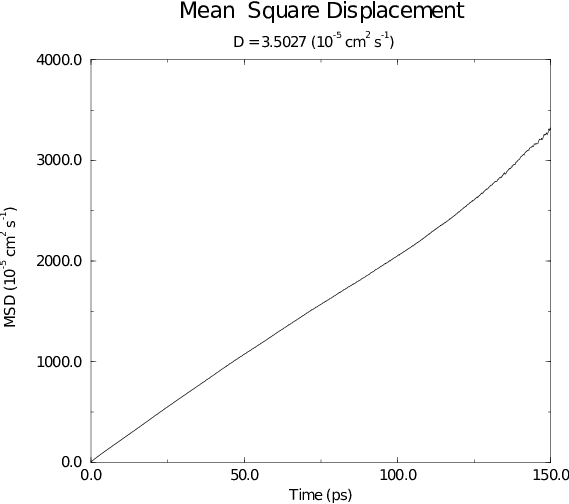# Mean Square Displacement¶

To determine the self diffusion coefficient $$D_A$$ of particles of type $$A$$, one can use the Einstein relation 108:
(1)$\lim_{t \rightarrow \infty} \langle \|{\bf r}_i(t) - {\bf r}_i(0)\|^2 \rangle_{i \in A} ~=~ 6 D_A t$
This mean square displacement and $$D_A$$ are calculated by the program gmx msd. Normally an index file containing atom numbers is used and the MSD is averaged over these atoms. For molecules consisting of more than one atom, $${\bf r}_i$$ can be taken as the center of mass positions of the molecules. In that case, you should use an index file with molecule numbers. The results will be nearly identical to averaging over atoms, however. The gmx msd program can also be used for calculating diffusion in one or two dimensions. This is useful for studying lateral diffusion on interfaces.

An example of the mean square displacement of SPC water is given in Fig. 53.Fig. 53 Mean Square Displacement of SPC-water.